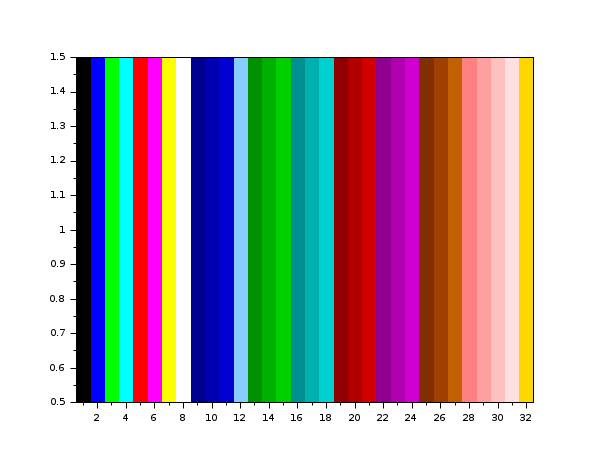Scilab Home page | Wiki | Bug tracker | Forge | Mailing list archives | ATOMS | File exchange
Change language to: Français - Português - 日本語 - Русский
Scilab Help >> Graphics > 2d_plot > Matplot

# Matplot

2D plot of a matrix using colors

### Syntax

```Matplot(a, [strf, rect, nax])
Matplot(a, <opt_args>)```

### Arguments

a

a real matrix of size (`n1`, `n2`).

<opt_args>

this represents a sequence of statements `key1=value1, key2=value2, ...` where `key1`, `key2`, ... can be one of the following:

rect

sets the bounds of the plot. If this key is given and neither `frameflag` nor `strf` is given then the `y` character of `strf` is supposed to be `7`. See below for value.

nax

sets the grids definition. If this key is given and neither `axesflag` nor `strf` is given then the `z` character of `strf` is supposed to be `1`. See below for value.

frameflag

specifies how the frame of the plot is computed. The value is an integer ranging from `0` to `8`. It corresponds to the `y` character of `strf`. See below.

axesflag

specifies what kind of axes are drawn around the plot. The value is an integer ranging from `0` to `5`. It corresponds to the `z` character of `strf`. See below.

strf

is a string of length 3 `"xyz"`.

default

the default is `"081"`.

x

controls the display of captions.

x=0

no caption.

x=1

captions are displayed. They are given by the optional argument `leg`.

y

controls the computation of the actual coordinate ranges from the minimal requested values. Actual ranges can be larger than minimal requirements.

y=0

no computation, the plot use the previous (or default) scale.

y=1

from the `rect` argument.

y=2

from the min/max of the x, y data.

y=3

built for an isometric scale from the `rect` argument.

y=4

built for an isometric plot from the min/max of the x, y data.

y=5

enlarged for pretty axes from the `rect` argument.

y=6

enlarged for pretty axes from the min/max of the x, y data.

y=7

like `y=1` but the previous plots are redrawn to use the new scale.

y=8

like `y=2` but the previous plots are redrawn to use the new scale.

z

controls the display of information on the frame around the plot. If axes are requested, the number of ticks can be specified by the `nax` optional argument.

z=0

nothing is drawn around the plot.

z=1

axes are drawn, the y-axis is displayed on the left.

z=2

the plot is surrounded by a box without ticks.

z=3

axes are drawn, the y-axis is displayed on the right.

z=4

axes are drawn centred in the middle of the frame box, with the box disabled.

z=5

axes are drawn centred in the middle of the frame box, with the box enabled.

rect

This argument is used when the second character `y` of argument `strf` is `1`, `3` or `5`. It is a row vector of size 4 and gives the dimension of the frame: `rect = [xmin, ymin, xmax, ymax]`.

nax

This argument is used when the third character `z` of argument `strf` is `1`. It is a row vector with four entries `[nx, Nx, ny, Ny]` where `nx` (`ny`) is the number of subgraduations on the x (y) axis and `Nx` (`Ny`) is the number of graduations on the x (y) axis.

### Description

The entries of matrix `int(a)` are used as colormap entries in the current colormap. The color associated to `a(i,j)` is used to draw a small square of size 1 with center at location `(x=j, y=(n1-i+1))`. If a matrix entry is outside the colormap, the corresponding rectangle is not displayed.To update the Matplot data, the `data` field of the corresponding handle must be used.
`data` can be a matrix (or an hypermatrix) containing RGB, RGBA, ... data (see Matplot_properties).

Enter the command `Matplot()` to see a demo.

### Examples

`Matplot([1 2 3;4 5 6])````clf()
// draw the current colormap
nbcol = size(gcf().color_map, 1);
Matplot(1:nbcol)```### History

 Version Description 5.5.0 `data` can now be a matrix (or an hypermatrix) containing RGB, RGBA, ... data (see Matplot_properties).